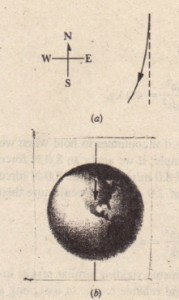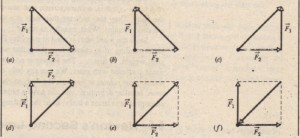# Force

Force

We now wish to define the unit of force. We know that a force can cause the acceleration of a body. Thus, we shall define the unit of force in terms of the acceleration that a force gives to a standard reference body. As the standard body, we shall use (or rather imagine that we use) the standard kilogram. This  body has been assigned, exactly and by definition, a mass of 1 kg.

Thus, a force is measured by the acceleration it produces. However, acceleration is a vector quantity, with both magnitude and direction. Is force also a vector quantity? We can easily assign a direction to a force (just assign the direction of the acceleration), but that is not sufficient. We must prove by experiment that forces are vector quantities. Actually, that has been done: forces are indeed vector quantities;they have magnitudes and directions and they combine according to the vector rules of Chapter 3.

In this book, forces are most often represented with a vector symbol such as , and a net force is represented with the vector symbol.As with other vectors, a force or a net force can have components along coordinate axes. When forces act along a single axis, they are single-component forces. Then we can drop the overhead arrows the force symbols and just use signs to indicate the directions of the forces along that axis.

Newton’s First Law: If net  force for  body , then the body’s velocity cannot change that is the body cannot accelerate.

There may be multiple forces acting on a body, but if their net (or resultant) force is zero, then the body cannot accelerate.

Inertial Reference Frames

Newton’s first law is not true in all reference frames: but we can always find reference frames in which it (and the rest of Newtonian mechanics) is true. Such frames are called inertial reference frames, or simply inertial frames.

An inertial reference frame is one in which Newton’s laws hold.

For example, we can assume that the ground is an inertial frame provided that we can neglect Earth’s actual astronomical motions (such as its rotation).

That assumption works well if we, say, send a puck sliding along a short trip of friction less ice-s-an observer on the ground would find that the puck’s motion obeys the laws of Newtonian mechanics. However, suppose we made the strip very  long, extending, say, from north to south. Then an observer on the ground would  find that the puck accelerates slightly toward the west as it moves south .

In this book we usually assume that the ground is an inertial frame and that
measurements of forces and accelerations are made from it. If measurements are made in, say, an elevator that is accelerating relative to the ground them the measurements are being made in a non inertial frame and the results can be surprising.(0) The path of a puck that issent sliding due south along a longstrip of frictionless ice, as seen by anobserver on the ground. (b) The ground beneath the southward slidingpuck rotates to the east as Earth rotates.

CHECKPOINT 1: Which of the figure’s six arrangements correctly show the vector addition of forces 1, and 12 to yield the third vector, which is meant to represent their net  force .